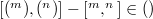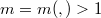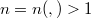﻿

### On rings and Banach algebras with skew derivations

#### Abstract

In the present paper, we investigate the commutativity of a prime Banach algebra with skew derivations and prove that if$\Aa$ is prime Banach algebra and$\Aa$ has a nonzero continuous linear skew derivation$\f$ from$\Aa$ to$\Aa$ such that$[\f(\xa^{m}), \f(\ya^{n})] - [\xa^{m}, \ya^{n}] \in \z(\Aa)$ for an integers$m = m(\xa, \ya)>1$ and$n = n(\xa, \ya)>1$ and sufficiently many$\xa, \ya$, then$\Aa$ is commutative.

DOI Code: 10.1285/i15900932v40n1p73

Keywords: Prime Banach algebra; skew derivation

Full Text: PDF